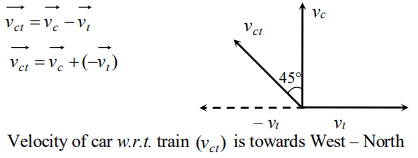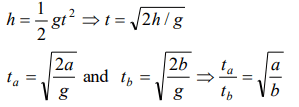## Motion in a Straight Line Questions and Answers Part-13

1. A river is flowing from W to E with a speed of 5 m/min. A man can swim in still water with a velocity 10 m/min. In which direction should the man swim so as to take the shortest possible path to go to the south
a) 30° with downstream
b) 60° with downstream
c) 120° with downstream
d) 150° with downstream

Explanation:2. A train is moving towards east and a car is along north, both with same speed. The observed direction of car to the passenger in the train is
a) East-north direction
b) West-north direction
c) South-east direction
d) None of these

Explanation:3. An express train is moving with a velocity v1. Its driver finds another train is moving on the same track in the same direction with velocity v2. To escape collision, driver applies a retardation a on the train. the minimum time of escaping collision will be
a) $t=\frac{v_{1}-v_{2}}{a}$
b) $t_1=\frac{v_1^2-v_2^2}{2}$
c) None
d) Both

Explanation:4. A stone falls from a balloon that is descending at a uniform rate of 12 m / s . The displacement of the stone from the point of release after 10 sec is
a) 490 m
b) 510 m
c) 610 m
d) 725 m

Explanation:5. A ball is dropped on the floor from a height of 10 m. It rebounds to a height of 2.5 m. If the ball is in contact with the floor for 0.01 sec, the average acceleration during contact is
a) $2100 m\diagup sec^{2}downwards$
b) $2100 m\diagup sec^{2}upwards$
c) $1400 m\diagup sec^{2}$
d) $700 m\diagup sec^{2}$

Explanation:6. A body A is projected upwards with a velocity of $98 m\diagup s$ . The second body B is projected upwards with the same initial velocity but after 4 sec. Both the bodies will meet after
a) 6 sec
b) 8 sec
c) 10 sec
d) 12 sec

Explanation:7. Two bodies of different masses $m_{a}$ and $m_{b}$ are dropped from two different heights a and b . The ratio of the time taken by the two to cover these distances are
a) $a:b$
b) $b:a$
c) $\sqrt{a}:\sqrt{b}$
d) $a^{2}:b^{2}$

Explanation:8. A body falls freely from rest. It covers as much distance in the last second of its motion as covered in the first three seconds. The body has fallen for a time of
a) 3 s
b) 5 s
c) 7 s
d) 9 s

Explanation:9. An iron ball and a wooden ball of the same radius are released from the same height in vacuum. They take the same time to reach the ground. The reason for this is
a) Acceleration due to gravity in vacuum is same irrespective of the size and mass of the body
b) Acceleration due to gravity in vacuum depends upon the mass of the body
c) There is no acceleration due to gravity in vacuum
d) In vacuum there is a resistance offered to the motion of the body and this resistance depends upon the mass of the body